Function Repository Resource:

# PlotVector

Plot a list of vectors in the plane

Contributed by: Dennis M Schneider
 ResourceFunction["PlotVector"][{{x1,y1},{x2,y2},…}] plots the vectors {xi,yi}, each located at the origin. ResourceFunction["PlotVector"][{{x1,y1},{x2,y2},…},{p,q}] plots the vectors {xi,yi}, each located at the point {p,q}. ResourceFunction["PlotVector"][{{{x1,y1},{p1,q1}},{{x2,y2},{p2,q2}},…}] plots the vectors {xi,yi} located at the corresponding points {pi,qi}. ResourceFunction["PlotVector"][{{x1,y1},{x2,y2},…},{{p1,q1},{p2,q2},…}] plots the vectors {xi,yi} located at the corresponding points {pi,qi}.

## Details and Options

ResourceFunction["PlotVector"] accepts the following options:
 "ArrowSize" Medium specify the size of the arrowhead "LocatedVector" False whether to draw vectors from the initial point (the first point of the list) to the terminal point (the second point in the list) VectorStyle RGBColor[.3,.4,.7] specify the style of the vector

## Examples

### Basic Examples (5)

Plot the vector (1,1) located at the origin:

 In:=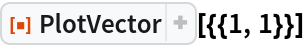Out=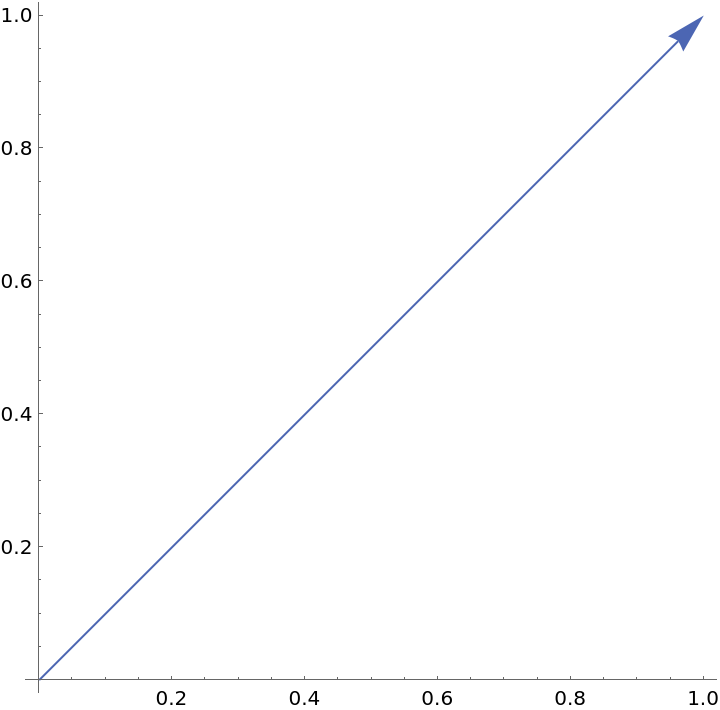Plot a list of vectors located at the origin:

 In:=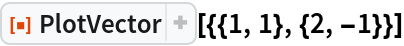Out=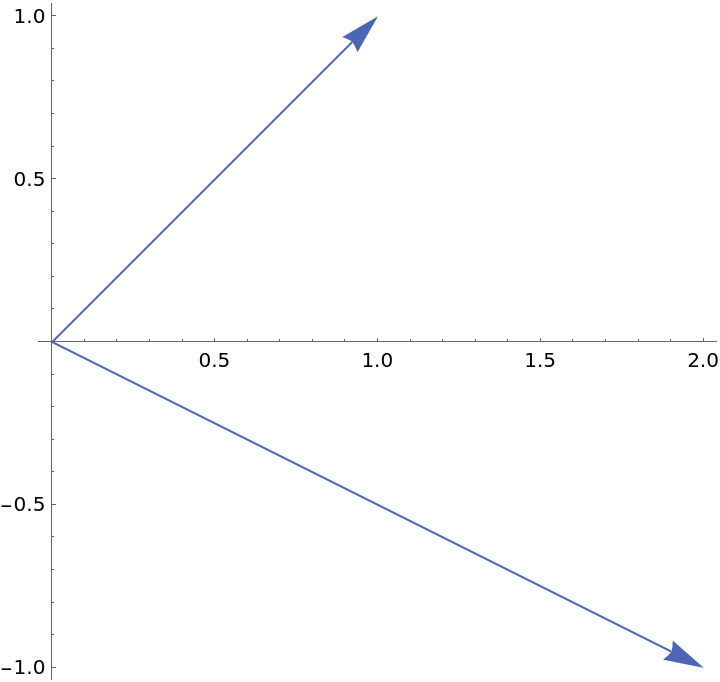Plot the vector (2,1) located at the point (-1,1):

 In:=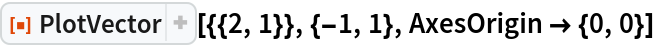Out=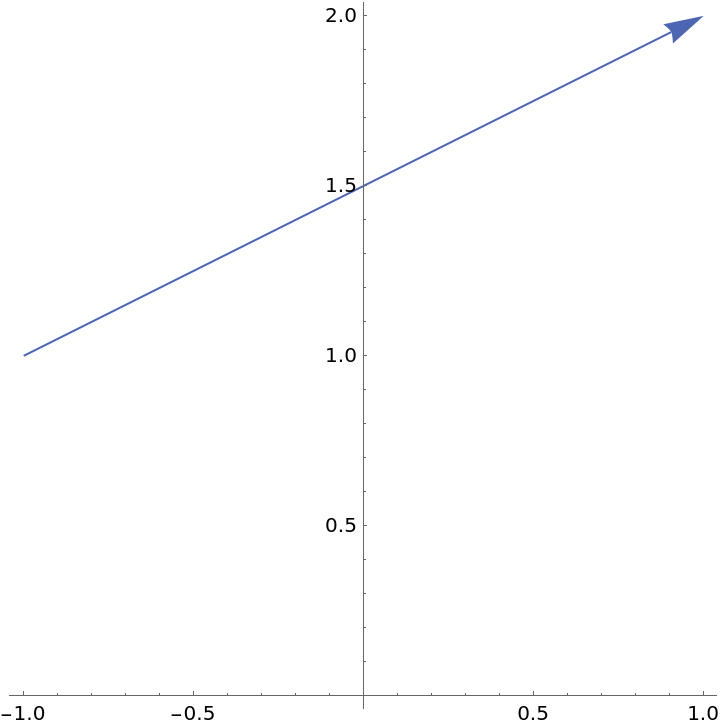Plot a list of vectors located at the point {1,2}:

 In:=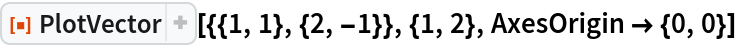Out=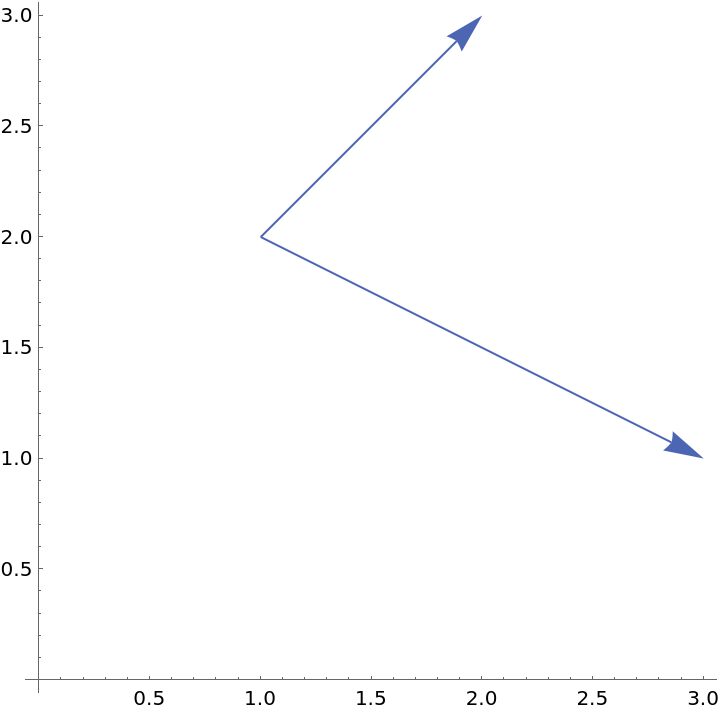Plot a list of vectors located at different points. The first list is a list of vectors and the second is a list of initial points:

 In:=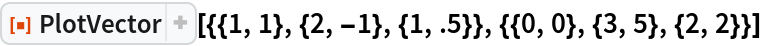Out=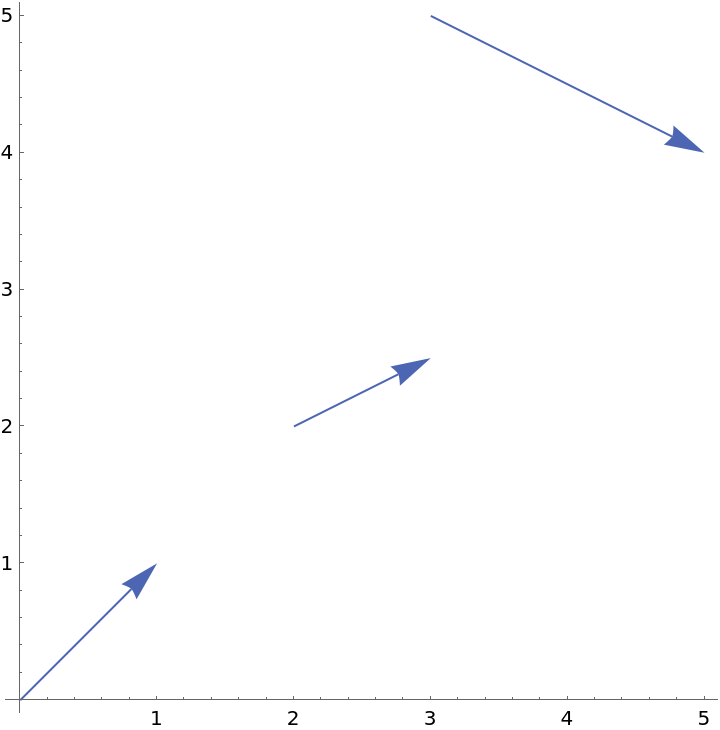### Scope (1)

Specify a vector located at a point in three different ways:

 In:=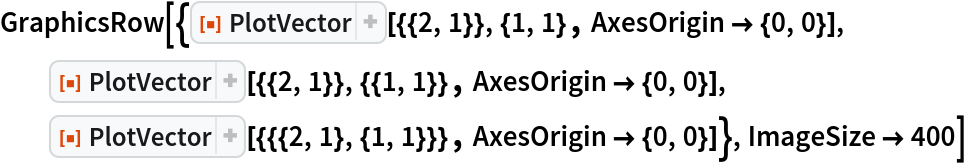Out=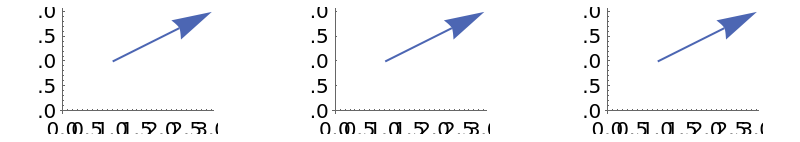### Options (6)

#### ArrowSize (2)

The default arrow size is Medium, which corresponds (roughly) to "ArrowSize"1.5:

 In:=Out=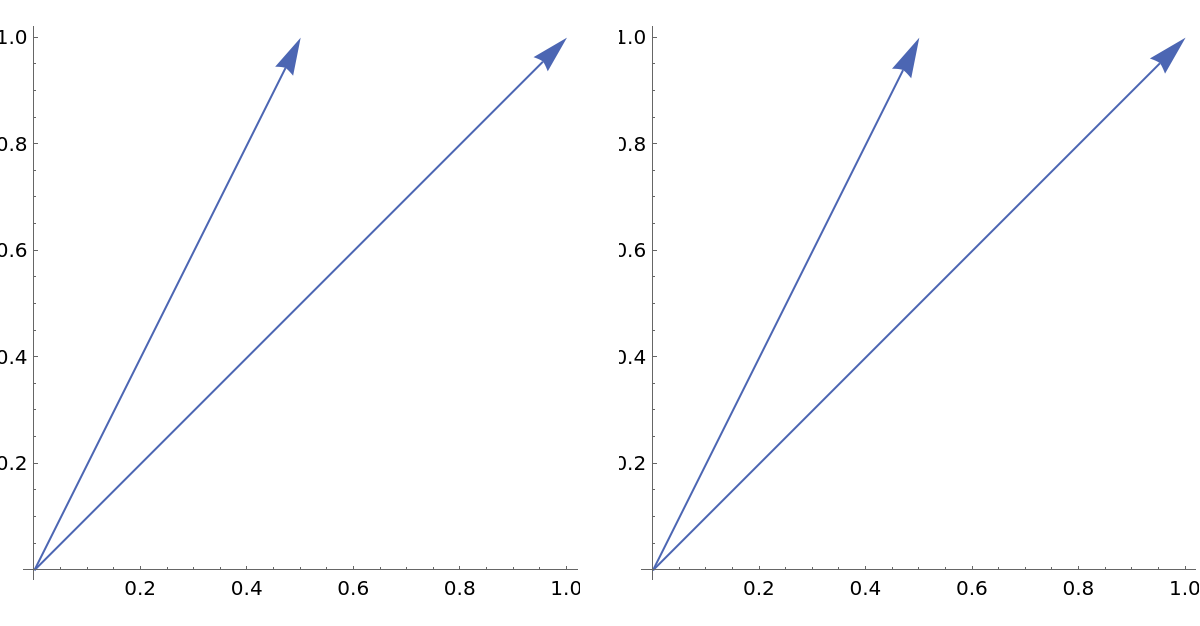Change the arrow size of each vector:

 In:=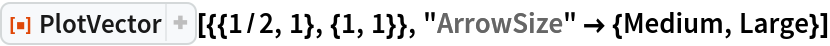Out=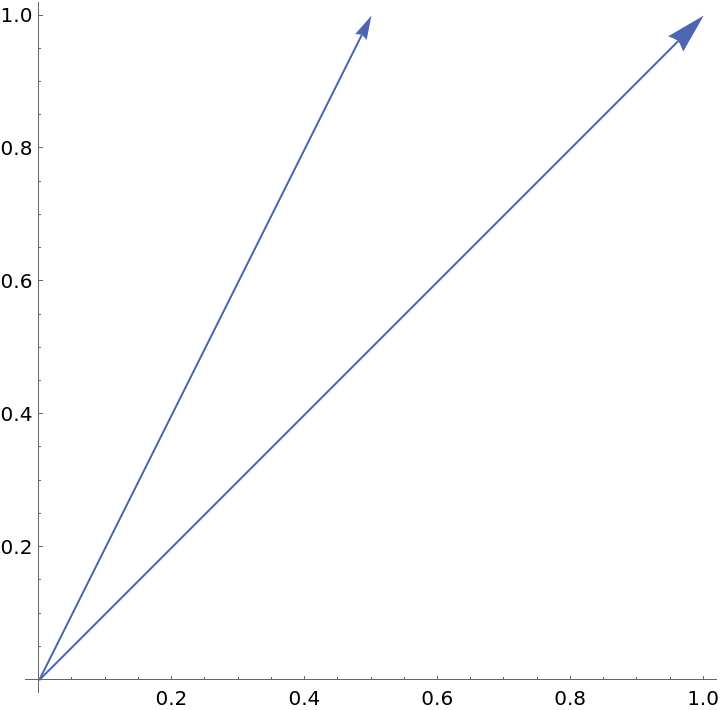#### LocatedVector (2)

A located vector from the first point to the second point:

 In:=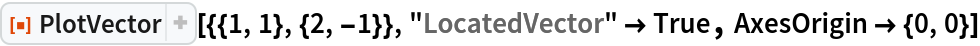Out=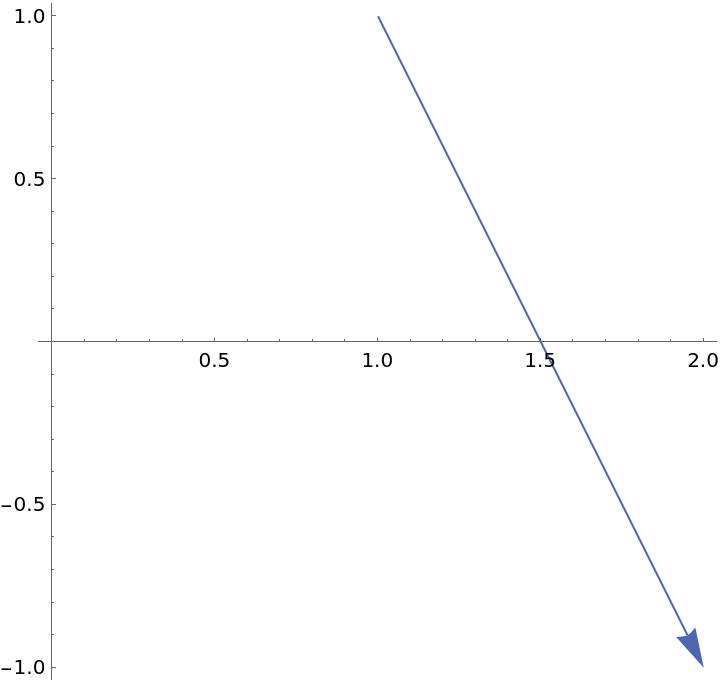Setting the located vector to False draws the vectors located at the origin:

 In:=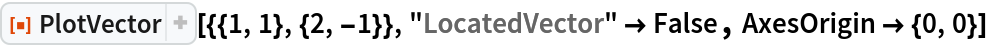Out=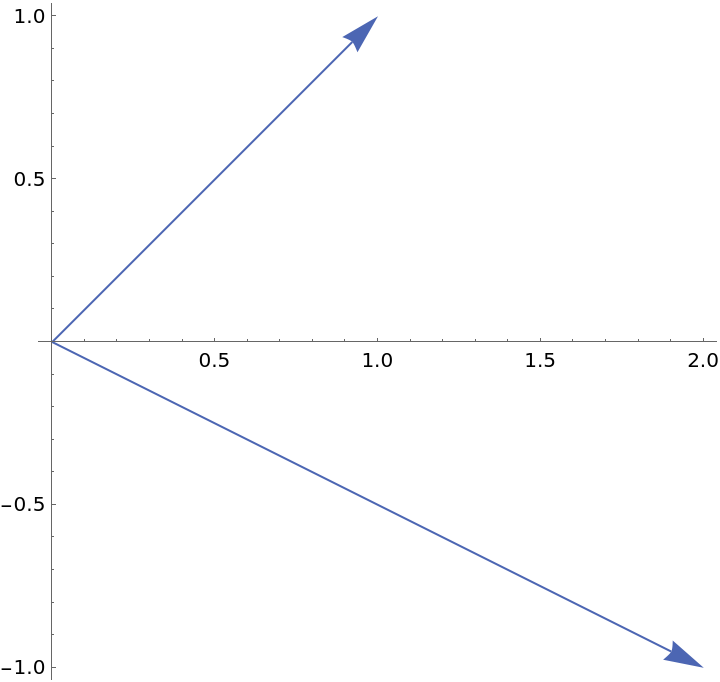A list of lists of pairs of vectors with the option "LocatedVector"True draws located vectors from the first point in each sublist to the second point in each sublist:

 In:=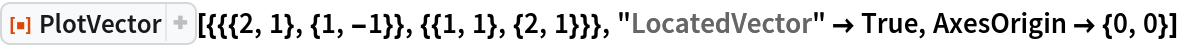Out=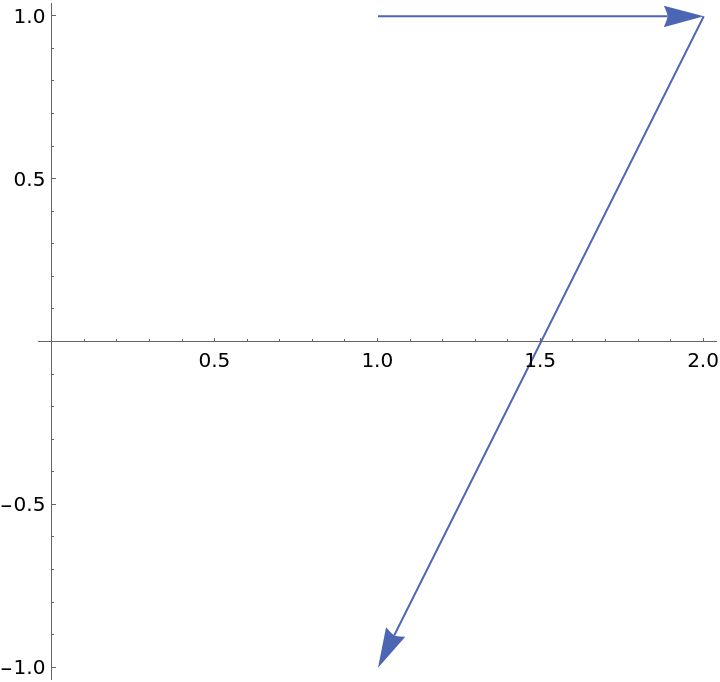Without the option being set, the second tuple in each list gives the located point, and the first tuple gives the direction:

 In:=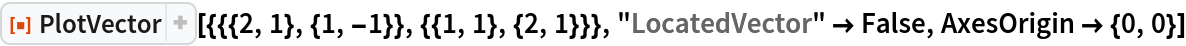Out=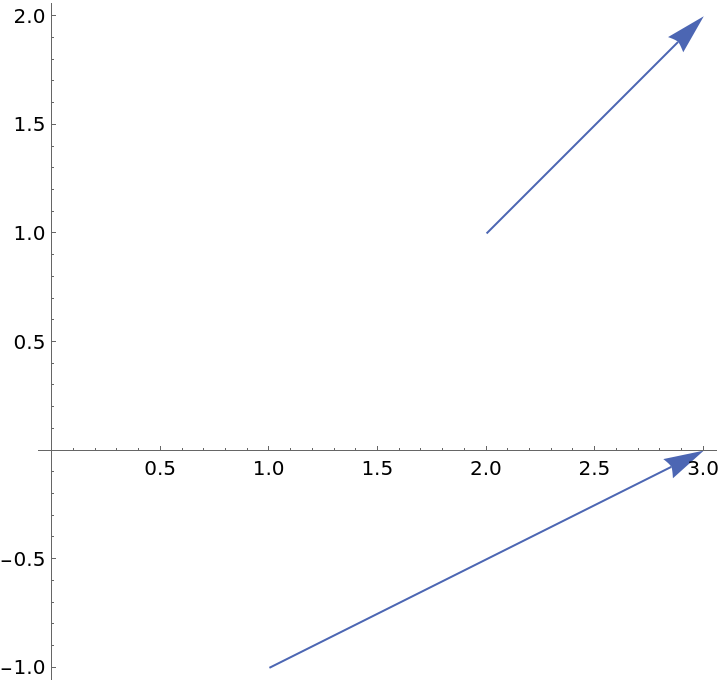#### VectorStyle (2)

Change the style of the vectors:

 In:=Out=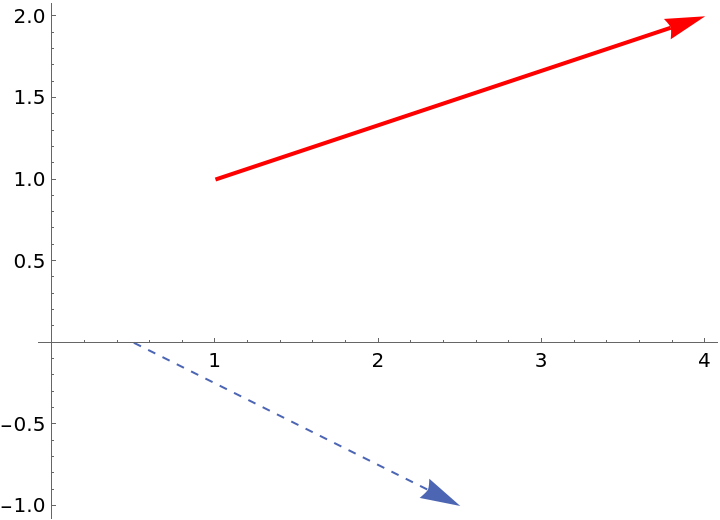Set both the arrow size and style for each vector:

 In:=Out=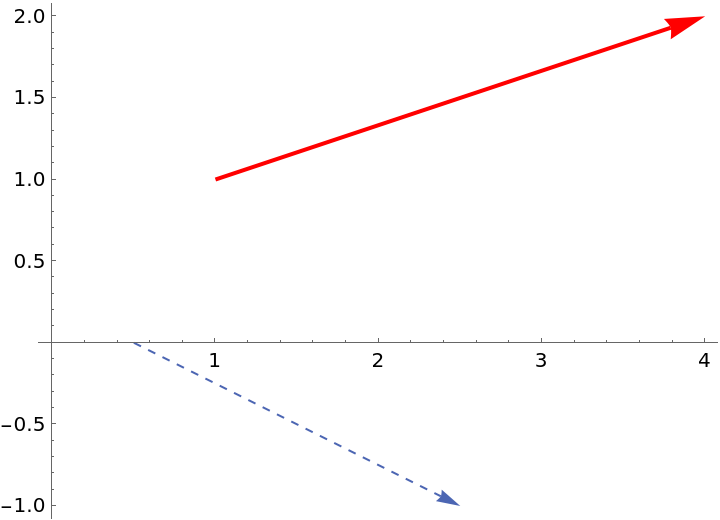### Possible Issues (1)

The length of the list of vectors must be the same as the length of the list of initial points:

 In:=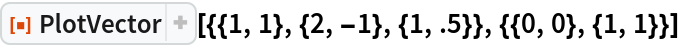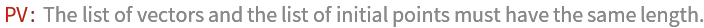## Publisher

Dennis M Schneider

## Version History

• 1.0.0 – 28 August 2019